# Test: Probability And Statistics - 2

## 20 Questions MCQ Test Topic-wise Tests & Solved Examples for IIT JAM Mathematics | Test: Probability And Statistics - 2

Description
Attempt Test: Probability And Statistics - 2 | 20 questions in 60 minutes | Mock test for Mathematics preparation | Free important questions MCQ to study Topic-wise Tests & Solved Examples for IIT JAM Mathematics for Mathematics Exam | Download free PDF with solutions
QUESTION: 1

### A problem is given to three students A,B and C whose chances of solving it are 1/2 ,1/3 and 1/4 respectively. The probability that this problem will be solved, is :

Solution:

Required probability = 1 - probability that no student solve the problem.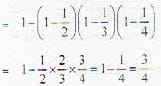QUESTION: 2

### 6 boys and 6 girls sit in a row randomly. The probability that all the girls sit together is :

Solution:

Required probability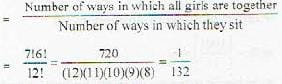QUESTION: 3

### Probabilities of three students A, B and C to pass an examination arc respectively 1/3,1/4 and 1/5. The probability that exactly one student will pass is :

Solution:

Required probability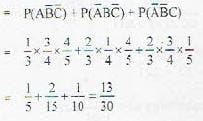QUESTION: 4

Different words are written with the letters of PEACE. The probability that both E’s come together is :

Solution:

Required probability =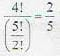QUESTION: 5

The probability of throwing 6 at least one in four throws of a die is :

Solution:

Probability of throwing 6 at least once = 1 - probability of not throwing 6.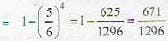QUESTION: 6

An untrue coin is such that, when it is tossed the chances of appearing head is twice the chances of appearance of tail. The chance of getting head in one toss of the coin is :

Solution:

If k is probability of occurrence of head., then k/2 will be probability of occurence of tail, so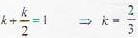QUESTION: 7

The probability of randomly choosing 3 defectless bulbs from 15 electric bulbs of which 5 bulbs are defective, is :

Solution:

Probability required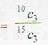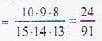QUESTION: 8

Probability of four digit numbers, which are divisible by three, formed out of digits 1,2,3,4.5 is :

Solution:

Four digit numbers formed with digits 1,2 .4 ,5 will be divisible by three, so required probability is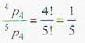QUESTION: 9

If X is uniformly distributed over (0, 10), the probability that 1 < X < 6 is

Solution:

Probability density function of X which is uniformly distributed in (0, 10) is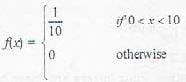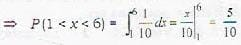QUESTION: 10

The choice of throwing 12 in a single throw with three dice is

Solution:

If the numbers appearing on the face of dice are such that the sum of the values on their faces lesser than 6 is equal to 6, with the constraint that 6 is not subtracted from a single dice. So, required probability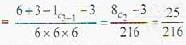QUESTION: 11

X is a continuous random variable with probability’ function f(x) = N exp(-x2+6x) - ∝ < x < ∝, the value of N is

Solution: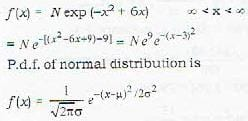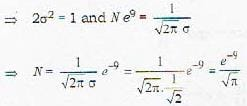QUESTION: 12

X is an exponential random variable with parameter λ with p.d.f. (x) = λe-7x if x ≥ 0 = 0 if x < 0, identify the correct one

Solution: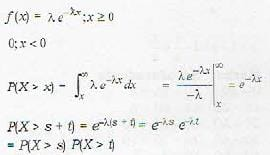QUESTION: 13

Determine the value of K for which the function given by f [x, y) = kxy for x = 1, 2, 3 and y = 1,2, 3 can serve as a joint probability distribution

Solution: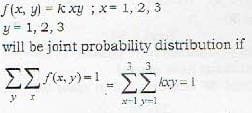⇒ K(1 + 2 + 3) (1 + 2 + 3) = 1 ⇒ k = 1/36

QUESTION: 14

On your way to work, you have to drive through a busy juction where you may be stopped at traffic lights. The cycle of the traffic light is 2 minutes of green followed by 3 minutes of red. What is the expected delay in the journey, if you arrive at the junction at a random time uniformly distributed over the whole 5 minute cycle is?

Solution:

Delay in journey is denoted by x then its probability density function.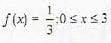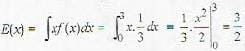QUESTION: 15

Let X have the poission distribution with parameter λ such that P(X = k - 1) = r(k) P(X = K). Then r[K] is

Solution: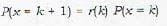⇒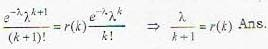QUESTION: 16

If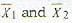are the means of two distributions such that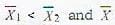is the mean of the combined distribution, then

Solution:

If nelements has mean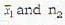elements has mean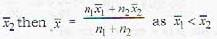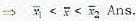QUESTION: 17

Suppose that the expected number of accidents per week at an industrial plant is 4. The number of workers injured in each accident is independent random variable with a common mean of 2. Assume also that the number of workers injured in each accident is independent of the number of accidents that occur. The expected number of injuries during a week is

Solution:

E(xy) = E(x) E(Y) = 4 x 2 = 8

QUESTION: 18

Let X and Y be independent random variables μand μy and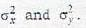Then Var (XY) equals

Solution: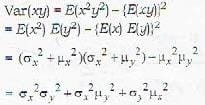QUESTION: 19

f(x) = k, exp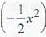for all x ∈ R; can be a probability density function for

Solution: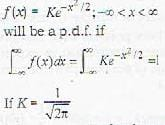it becomes standard normal distribution.

QUESTION: 20

Let x and y be independent random variables with binomial distribution B(10, 1 /3) and B(20, 1/3) respectively. E[x + y] is

Solution:

E (x + y) = E (x) + E(y)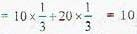Expectation of binomial distribution is np if B(n, p) is the binomial distribution.Use Code STAYHOME200 and get INR 200 additional OFF Use Coupon Code